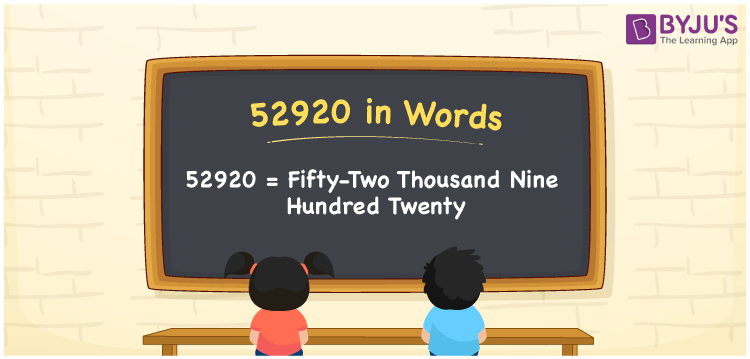# 52920 in Words

We can write 52920 in words as Fifty-two thousand nine hundred twenty. If you spent Rs. 52920 for the renovation of a hut, you can say, “I spent Fifty-two thousand nine hundred twenty rupees to renovate the hut”. Let’s learn how to write the cardinal number 52920 in word form with the help of a place value chart.

 52920 in words Fifty-two thousand nine hundred twenty Fifty-two thousand nine hundred twenty in Numbers 52920

## 52920 in English words

We generally write numbers in words using the English alphabet. Thus, we spell 52920 in English as “Fifty-two thousand nine hundred twenty”.## How to Write 52920 in Words?

Below is the place value chart for the number 52920, which is used to convert the number 52920 into words.

 Ten thousands Thousands Hundreds Tens Ones 5 2 9 2 0

Here, ones = 0, tens = 2, hundreds = 9, thousands = 2, ten thousands = 5.

By expanding these numbers based on their place values, we get;

5 × Ten thousand + 2 × Thousand + 9 × Hundred + 2 × Ten + 0 × One

= 5 × 10000 + 2 × 1000 + 9 × 100 + 2 × 10 + 0 × 1

= 50000 + 2000 + 900 + 20

= Fifty thousand + Two thousand + Nine hundred + Twenty

= Fifty-two thousand nine hundred twenty

Therefore, 52920 in words = Fifty-two thousand nine hundred twenty.

52920 is a natural number that is the successor of 52919 and the predecessor of 52921.

52920 in words – Fifty-two thousand nine hundred twenty

Is 52920 an odd number? – No

Is 52920 an even number? – Yes

Is 52920 a perfect square number? – No

Is 52920 a perfect cube number? – No

Is 52920 a prime number? – No

Is 52920 a composite number? – Yes

## Frequently Asked Questions on 52920 in Words

Q1

### What is 52920 in word form?

Fifty-two thousand nine hundred twenty is the word form of the number 52920.
Q2

### How to write Rs. 52920 in words on a cheque?

On a cheque, we generally write Rs. 52920 in words as Fifty-two thousand nine hundred twenty rupees only.
Q3

### Express the value of 52920 + 4080 in words.

52920 + 4080 = 57000 Therefore, the value of 52920 + 4080, i.e., 57000 in words is Fifty-seven thousand.